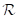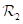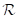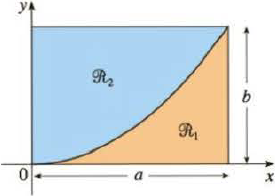Chapter 8.3, Problem 42E

Chapter
Section
Textbook Problem

A rectanglewith sides a and b is divided into two partsandby an arc of a parabola that has its vertex at one corner ofand passes through the opposite corner. Find the centroids of bothand.To determine

To find: The centroid of both part in the rectangle R1 and R2.

Explanation

Given:

The length of the rectangle is a.

The width of the rectangle is b.

Calculation:

Draw the rectangular as shown in Figure 1.

Refer to Figure 1.

The part in the rectangle (R1) is parabola‑1.

The part in the rectangle (R2) is parabola‑2.

Consider the area (A) of the rectangle (R) is,

A=ab

The equation of parabola is as shown,

y=kx2 (1)

Substitute a for x and b for y in Equation (1).

b=ka2k=ba2

Substitute ba2 for k in Equation (1).

y=ba2x2

Calculate the area (A1) of the parabola‑1:

A1=abydx (2)

Substitute 0 for a, a for b, and ba2x2 for y in Equation (2).

A1=0aba2x2dx=ba20ax2dx (3)

Integrate Equation (3).

A1=ba2[x2+12+1]0a=ba2[x33]0a=ba2(a330)=ab3

Calculate the (x¯1) coordinate of centroid of the parabola‑1:

x¯1=1A1abxydx (4)

Substitute 0 for a, a for b, ab3 for A1, and ba2x2 for y in Equation (4).

x¯1=1ab30ax(ba2x2)dx=3ab×ba20ax3dx=3a30ax3dx (5)

Integrate Equation (5).

x¯1=3a3[x3+13+1]0a=3a3[x44]0a=3a3(a440)=34a

Calculate the (y¯1) coordinate of centroid of the parabola‑1:

y¯1=1A1ab12[f(x)]2dx (6)

Substitute 0 for a, a for b, ab3 for A1, and ba2x2 for y in Equation (6).

y¯1=1ab30a12(ba2x2)2dx=3ab×12×b2a40ax4dx=3b2a50ax4dx (7)

Integrate Equation (7).

y¯1=3b2a5[x4+14+1]0a=3b2a5[x55]0a=3b2a5(a550)=310b

Hence, the centroid of part in the rectangle (R1) is (34a,310b)_.

Calculate the area (A2) of the parabola‑2:

A=A1+A2 (8)

Substitute ab for A, and ab3 for A1 in Equation (8)

Still sussing out bartleby?

Check out a sample textbook solution.

See a sample solution

The Solution to Your Study Problems

Bartleby provides explanations to thousands of textbook problems written by our experts, many with advanced degrees!

Get Started

Expand each expression in Exercises 122. (2x3)2

Finite Mathematics and Applied Calculus (MindTap Course List)

In problems 23-58, perform the indicated operations and simplify. 52.

Mathematical Applications for the Management, Life, and Social Sciences

For y=12gt2 (where g is a constant), dydt=. a) 14g2 b) 12g c) gt d) gt2

Study Guide for Stewart's Single Variable Calculus: Early Transcendentals, 8th

True or False: converges mean exists.

Study Guide for Stewart's Multivariable Calculus, 8th

Evaluate each sum. k=15(3k1)

College Algebra (MindTap Course List)

Describe how replication protects against fraud being committed in research.

Research Methods for the Behavioral Sciences (MindTap Course List)